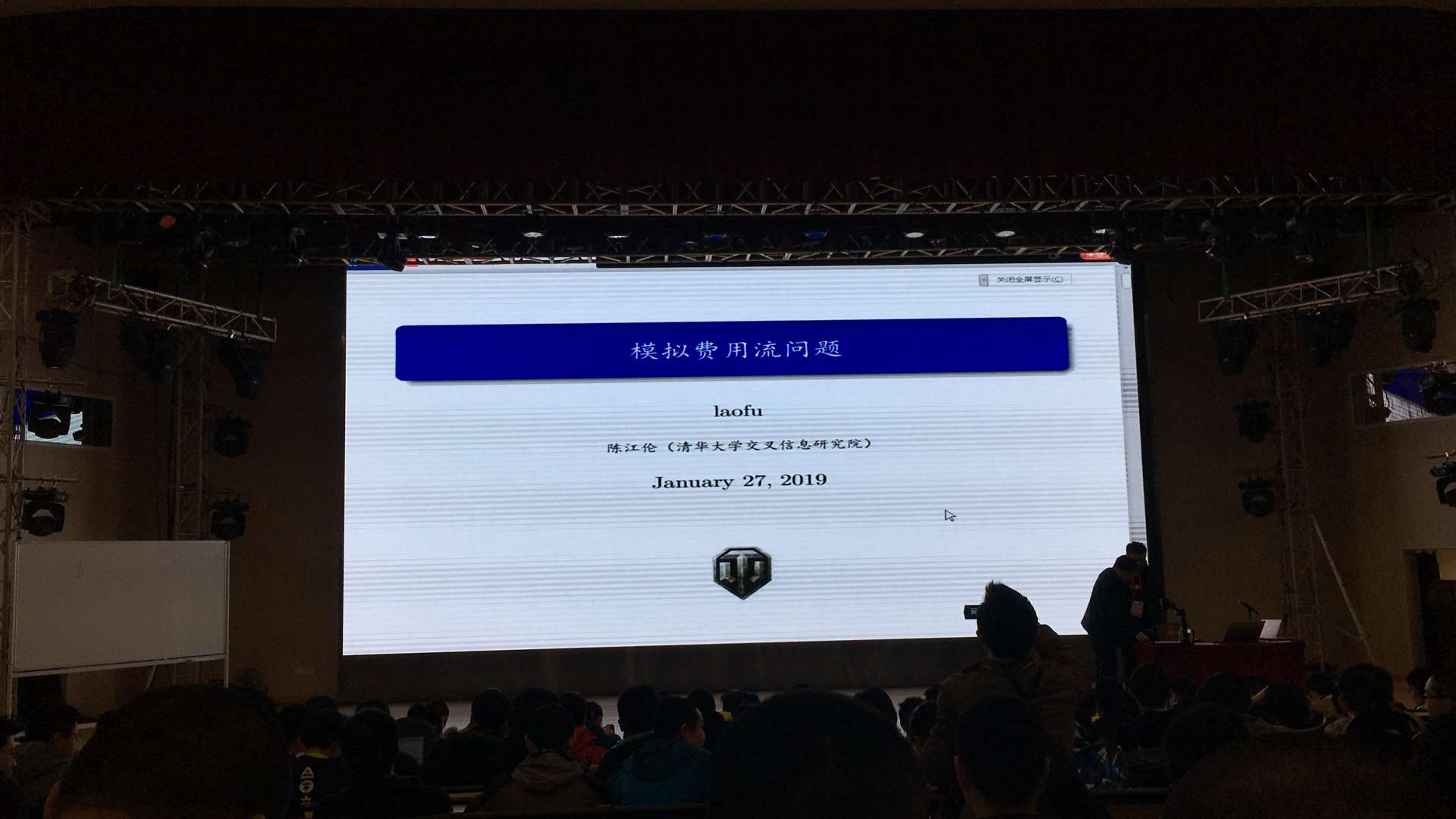# 定语从句

## 简介

Codeforces是一个由俄罗斯萨拉托夫州立大学建立并维护的算法竞赛网站，优势在于其频繁且高质量的在线比赛。

## 赛制

Codeforces自创了一套独特的赛制。这套赛制类似于标准的ACM赛制，但是相比ACM赛制又更加的多样化。

### 积分

≥ 3000黑色&红色Legendary Grandmaster
2600 — 2999红色International Grandmaster
2400 — 2599红色Grandmaster
2300 — 2399橙色International Master
2100 — 2299橙色Master
1900 — 2099紫色Candidate Master
1600 — 1899蓝色Expert
1400 — 1599青色Specialist
1200 — 1399绿色Pupil
≤ 1199灰色Newbie

# 2019暑假打过的网络赛

Codeforces Round #574 (Div. 2)
AtCoder Beginner Contest 134
Codeforces Global Round 4

## 集训机构藏身广州，打着学有余力的旗号，各高校如何回应？## 从早八点学到晚九点

### 后排学生只能坐二楼## A. Drinks Choosing 2s/256MB

### 题意

``````#include <iostream>

const int N = 1001;

int cnt[N];

int main () {
register int i, j;
int n, m, x;

std::cin >> n >> m;
for (i = 1; i <= n; ++ i) {
std::cin >> x;
++ cnt[x];
}

int ans = 0;
for (i = 1; i <= m; ++ i) {
ans += (cnt[i] % 2);
}

std::cout << n - ans / 2;

return 0;
}
``````

## B. Sport Mafia 2s/256MB

### 解法

``````#include <iostream>
#include <cmath>

typedef long long ll;

int main () {
ll n, k, m, d;

std::cin >> n >> k;
m = (n + k) * 2;
d = std::round(std::sqrt(9 + 4 * m));
std::cout << n - (d  - 3) / 2 << "\n";

return false;
}``````

### 解法

``````#include <iostream>

typedef long long ll;
const int N = 1e5+1;

ll a[N], f[N];

inline ll max(ll x, ll y) {
return x > y ? x : y;
}

int main () {
register int i, j;
ll n;

std::cin >> n;
for (i = 1; i <= n; ++ i) std::cin >> a[i];
for (i = 1; i <= n; ++ i) std::cin >> a[i];

for (i = 1; i <= n; ++ i) {
f[i] = max(f[i - 1], f[i - 1]) + a[i];
f[i] = max(f[i - 1], f[i - 1]) + a[i];
f[i] = max(f[i - 1], max(f[i - 1], f[i - 1]));
}

std::cout << max(f[n], max(f[n], f[n]));

return 0;
}``````